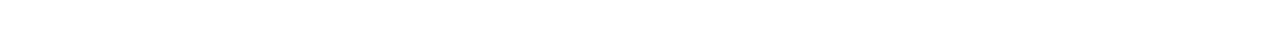# 26.1.2 Mean Power

### Mean Power

• In mains electricity, current and voltage are varying all the time
• This also means the power varies constantly, recall the equations for power:• Where:
• I = direct   current (A)
• V = direct voltage (A)
• R = resistance (Ω)
• The r.m.s values means equations used for direct current and voltage can now be applied to alternating current  and voltage
• They are also used determining an average current or voltage for alternating supplies
• Recall the equation for peak current:Step 3:            Calculate the mean power

• The mean power is half of the maximum (peak) power

Mean power = 1440 / 2 = 720 W### Author: Katie

Katie has always been passionate about the sciences, and completed a degree in Astrophysics at Sheffield University. She decided that she wanted to inspire other young people, so moved to Bristol to complete a PGCE in Secondary Science. She particularly loves creating fun and absorbing materials to help students achieve their exam potential.
CloseClose